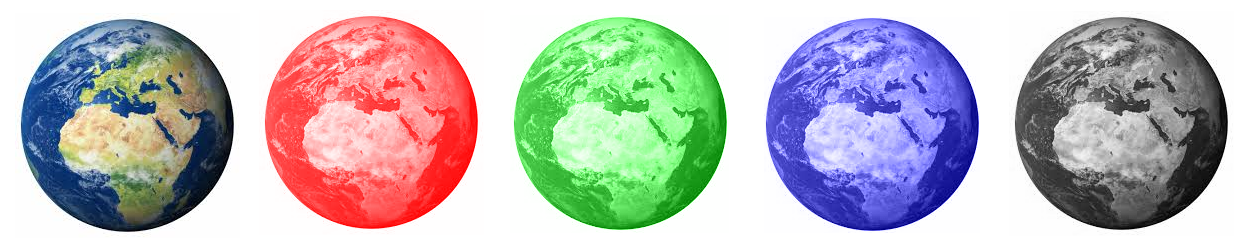# Basic color scale conversion algorithm

Your app has some pictures/icons, and you’d like to have them in different color scales, for highlighting for example.
Rather than creating a different set of icons, it is cheaper to convert them dynamically to the desired color : redscale, greenscale, or bluescale.The greyscale conversion algorithm is pretty straightforward: for each pixel of the picture, compute the average of its 3 colors (red, green, blue), and assign it to each color :

1. for each pixel p
2. x = average(p.red, p.green, p.blue)
3. p.red = p.green = p.blue = x

The redscale/greenscale/bluescale conversion algorithm is almost identical. In addition to the previous steps, just reinforce the color that you’re interested in. Ex : for a redscale, C being the amount of color reinforcement value :

1. for each pixel p
2. x = average(p.red, p.green, p.blue)
3. p.green = p.blue = x
4. p.red = x + C

Below is a C++ builder implementation of the algo :

[code lang=cpp]
typedef enum
{
GreyScale,
BlueScale,
RedScale,
GreenScale
} ColorScaleMode;

void ColorScale(Graphics::TBitmap* bitmap, ColorScaleMode mode, byte colorReinforcement = 50)
{
if (bitmap == NULL)
return;

bitmap->PixelFormat = pf24bit;
for (int x = 0; x < bitmap->Width; x++)
{
for (int y = 0; y < bitmap->Height; y++)
{
TColor pixelColor = bitmap->Canvas->Pixels[x][y];
byte R = GetRValue(pixelColor);
byte G = GetGValue(pixelColor);
byte B = GetBValue(pixelColor);
byte average = (byte)(((int)R + (int)G + (int)B) / 3);
switch (mode)
{
case RedScale:
R = (byte)std::min((int)average + colorReinforcement, 255);
G = B = average;
break;
case GreenScale:
G = (byte)std::min((int)average + colorReinforcement, 255);
R = B = average;
break;
case BlueScale:
B = (byte)std::min((int)average + colorReinforcement, 255);
R = G = average;
break;
case GreyScale:
default:
B = R = G = average;
}
bitmap->Canvas->Pixels[x][y] = TColor(RGB(R, G, B));
}
}
}
[/code]